ASP & CSP Math – Chemical Equations 2019-03-08T17:01:31+00:00

# ASP & CSP Exam Math – Chemical and Industrial Hygiene

Both the ASP Safety Fundamentals Exam and the CSP (Certified Safety Professional) exam will include muliple questions regarding chemicals and industrial hygiene within the math portions of the exams. Here’s a brief overview of what to expect and a tutorial including a couple of solved examples to assist in your exam preparation.

## Corrosives

The acidity or alkalinity of a solution is characterized by the pH scale, which ranges from 0 to 14. pH is the “power of hydrogen” in a substance. Solutions with a pH of 7 are considered to be neutral, while any pH greater than 7 is a base (alkaline). Solutions with a pH of less than 7 are acidic, with acidity increasing with lower pH values.

The pH of a substance can be tested with litmus paper or a digital pH meter. When using litmus paper, the paper will turn red when exposed to a low (acidic) pH or blue when exposed to a high (base) pH.

A similar measurement, pOH, measures the alkalinity of a substance. pOH stands for “power of hydroxide.” pH and pOH always add together to equal 14.

Let’s work on how to calculate the pH of a solution, which is calculated by the formula:Where:
H+ = the concentration of Hydrogen ions

Similarly, the pOH of a solution can be calculated using the formula:Where:
OH- = is the concentration of hydroxide ions

Remember, the sum of the pH and pOH of a solution is always 14. So, if we are given that the pH = 4.5:Now, we can use these values to calculate the concentrations of hydrogen and hydroxide ions given the above formulas for pH and pOH: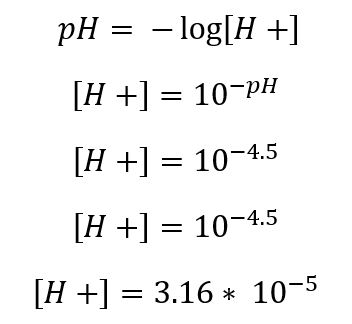## Flammables

Flammable liquids, such as gasoline, must be vaporized prior to burning, and airborne concentrations of vapors from a flammable liquid or flammable gases must be between the lower flammable limit (LFL) and upper flammable limit (UFL) to burn. As an example, gasoline has an LFL of 1.4% and a UFL of 7.6% and can only burn between those concentrations. Acetylene on the other hand has a much wider flammability range, with an LFL of 4.5% and a UFL of 81%. In this section, we’ll calculate the flammable range of a mixture of gases in the air through the use of LeChatelier’s mixing rule. The base formula is:Where:
UFLmix= upper flammability of the mixture
fn= fractional concentration of the component n
UFLn = upper flammability of the component n

The formula stays the same when we wish to solve for LFL instead of UFL, we simply substitute LFL into the equation: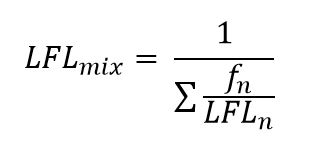Where:
LFLmix= lower flammability of the mixture
fn= fractional concentration of the component n
LFLn = lower flammability of the component n

Now, let’s apply the formula to solve for the UFL of a mixture of 50% methane (UFL 16.4%), 40% propane (UFL 10.1%) and 10% Hydrogen Sulfide (UFL 46%). The formula now looks like:

## Toxicity

Dose is a specific concentration or amount of a substance received across a period of time. This is represented by the formula:When discussing toxicity, dose is often expressed as an  LD50 or LC50, with is either the lethal dose (solids and liquids) or lethal concentration (gases) of a material that causes death in 50% of subjects who are exposed to it.

The World Health Organization (WHO) has developed a formula used to compare dioxins, one of the most toxic compounds in industry, to one another. Dioxins are the result of industrial processes, and unfortunately have been released into the natural environment throughout history, where they tend to accumulate and persist. Although there are hundreds of compounds that are grouped into dioxins, the most toxic is 2,3,7,8-TCDD. All other dioxins are compared to 2,3,7,8-TCDD by calculating their toxic equivalency factor (TEF). For example, a dioxin with a TEF of .25 would be one-quarter as toxic as the reference dioxin, 2,3,7,8-TCDD.

Often, compounds of dioxins are encountered. If we know the TEF of these dioxins and can determine percentages those dioxins make-up of the compound, we can use the following formula to determine the compound’s toxic equivalency (TEQ):Where:

TEQ = Toxic equivalency of a compound
mi= Concentration of each substance
TEFi= Toxic equivalency factor of each substance.

So, let’s work a problem. A dioxin compound has been found in the environment. Laboratory analysis indicates the compound is 60% Dioxin A, which has a TEF of .2, and 40% Dioxin B, which has a TEF of .5. What is the compound’s TEQ?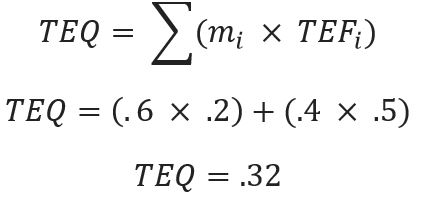## Balancing a Chemical Equation

The ability to balance a chemical equation will come in useful, especially for the ASP Safety Fundamentals exam, where you can expect to have at least 2 exam questions requiring you to balance chemical equations.

The law of conservation of mass states that for a system closed to transfers of matter and energy, the mass must remain constant over time, as mass cannot change quantity if it is not added or removed.

Because of this principle, chemical reactions are balanced in that the number of atoms of each type stays the same before and after a reaction. Below is an example of balancing a simple equation, similar to what may be seen on the exam. Let’s take a look at an unbalanced equation: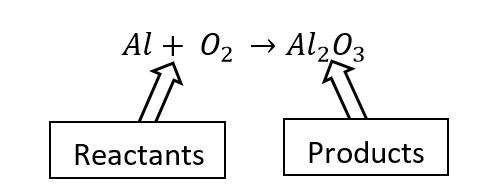Here’s an unbalanced equation in which Aluminum and Oxygen have reacted and combined to form Aluminum Oxide. The first thing we notice when we evaluate this equation is that the number of molecules on each side are not equal. All of a sudden we have 2 molecules of aluminum and 3 molecules of oxygen, when we started with just one molecule of aluminum and 2 molecules of oxygen. Due to the law of conservation of mass, this is impossible. We must have started with a different amount of oxygen and aluminum! Balancing the equation will help us figure that out.

Here’s our chemical equation again: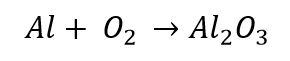Let’s multiple the reactants on the left so that our molecules equal come out balanced:Here we’ve multiplied the aluminum by two on the reactant side, and now it is equal to the molecules in the product. To get three oxygen molecules, we had to multiple by 1.5. This leaves us with 3 oxygen molecules (balanced), BUT there’s not really such a thing as 1.5 molecules of , so we have some work left to do. We need a whole number of molecules! Let’s use an algebraic principle to get there and multiply each side of the reaction by 2:That looks better, now we have 4 molecules of aluminum and 6 molecules of oxygen on each side of the chemical equation. The equation is balanced!

Let’s look at a slightly more complex chemical equation. In the equation below, we have ethylene and oxygen combining (actually this is combustion) on the reactant side. The combustion results in carbon dioxide and water forming, plus some heat being given off. The (g) in the equation just tells us the molecules are in the form of a gas. You’ll also see heat in the product. You can ignore / remove these if you’d like. Here’s the equation:You may find it helpful to draw a quick table to identify the imbalanced molecules:Let’s balance carbon first. We have 2 molecules in the reactant and 1 in the product, so simply multiply the  in the product by 2. If you’re using the chart, update it after each step!Now let’s look at the hydrogen. There’s 4 molecules in the reactants and still only 2 in the product. Multiply  H2O by two:We’re getting closer, now we can balance out our oxygen. Simply turn the single molecule of  in the reactants into 3 molecules:The equation is balanced. You may see the (g) and heat return in the answers.